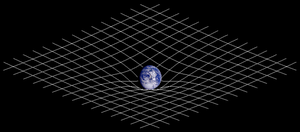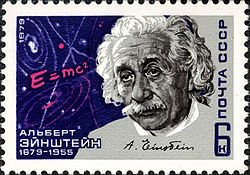# Theory of relativity

Theory of relativityTwo-dimensional projection of a three-dimensional analogy of spacetime curvature described in General Relativity

The theory of relativity, or simply relativity, encompasses two theories of Albert Einstein: special relativity and general relativity. However, the word relativity is sometimes used in reference to Galilean invariance.

The term "theory of relativity" was based on the expression "relative theory" (German: Relativtheorie) used by Max Planck in 1906, who emphasized how the theory uses the principle of relativity. In the discussion section of the same paper Alfred Bucherer used for the first time the expression "theory of relativity" (German: Relativitätstheorie).

## Scope

The theory of relativity enriched physics and astronomy during the 20th century. When first published, relativity superseded a 200-year-old theory of mechanics elucidated by Isaac Newton. It changed perceptions. However, Einstein denied that Newton could ever be superseded by his own work.

The theory of relativity overturned the concept of motion from Newton's day, by positing that all motion is relative. Time was no longer uniform and absolute. Physics could no longer be understood as space by itself, and time by itself. Instead, an added dimension had to be taken into account with curved spacetime. Time now depended on velocity, and contraction became a fundamental consequence at appropriate speeds.

In the field of physics, relativity catalyzed and added an essential depth of knowledge to the science of elementary particles and their fundamental interactions, along with ushering in the nuclear age. With relativity, cosmology and astrophysics predicted extraordinary astronomical phenomena such as neutron stars, black holes, and gravitational waves.

### Two theory view

The theory of relativity was representative of more than a single new physical theory. It affected the theories and methodologies across all the physical sciences. However, as stated above, this is more likely perceived as two separate theories. There are some explanations for this. First, special relativity was published in 1905, and the final form of general relativity was published in 1916.

Second, special relativity fits with and solves for elementary particles and their interactions, whereas general relativity solves for the cosmological and astrophysical realm (including astronomy).

Third, special relativity was widely accepted in the physics community by 1920. This theory rapidly became a significant and necessary tool for theorists and experimentalists in the new fields of atomic physics, nuclear physics, and quantum mechanics. Conversely, general relativity did not appear to be as useful. There appeared to be little applicability for experimentalists as most applications were for astronomical scales. It seemed limited to only making minor corrections to predictions of Newtonian gravitation theory. Its impact was not apparent until the 1930s.

Finally, the mathematics of general relativity appeared to be incomprehensibly dense. Consequently, only a small number of people in the world, at that time, could fully understand the theory in detail. This remained the case for the next 40 years. Then, at around 1960 a critical resurgence in interest occurred which has resulted in making general relativity central to physics and astronomy. New mathematical techniques applicable to the study of general relativity substantially streamlined calculations. From this physically discernible concepts were isolated from the mathematical complexity. Also, the discovery of exotic astronomical phenomena in which general relativity was crucially relevant, helped to catalyze this resurgence. The astronomical phenomena included quasars (1963), the 3-kelvin microwave background radiation (1965), pulsars (1967), and the discovery of the first black hole candidates (1971).

## On the theory of relativity

Einstein stated that the theory of relativity belongs to the class of "principle-theories". As such it employs an analytic method. This means that the elements which comprise this theory are not based on hypothesis but on empirical discovery. The empirical discovery leads to understanding the general characteristics of natural processes. Mathematical models are then developed which separate the natural processes into theoretical-mathematical descriptions. Therefore, by analytical means the necessary conditions that have to be satisfied are deduced. Separate events must satisfy these conditions. Experience should then match the conclusions.

The special theory of relativity and the general theory of relativity are connected. As stated below, special theory of relativity applies to all inertial physical phenomena except gravity. The general theory provides the law of gravitation, and its relation to other forces of nature.

## Special relativityUSSR stamp dedicated to Albert Einstein

Special relativity is a theory of the structure of spacetime. It was introduced in Einstein's 1905 paper "On the Electrodynamics of Moving Bodies" (for the contributions of many other physicists see History of special relativity). Special relativity is based on two postulates which are contradictory in classical mechanics:

1. The laws of physics are the same for all observers in uniform motion relative to one another (principle of relativity).
2. The speed of light in a vacuum is the same for all observers, regardless of their relative motion or of the motion of the source of the light.

The resultant theory agrees with experiment better than classical mechanics, e.g. in the Michelson-Morley experiment that supports postulate 2, but also has many surprising consequences. Some of these are:

• Relativity of simultaneity: Two events, simultaneous for one observer, may not be simultaneous for another observer if the observers are in relative motion.
• Time dilation: Moving clocks are measured to tick more slowly than an observer's "stationary" clock.
• Length contraction: Objects are measured to be shortened in the direction that they are moving with respect to the observer.
• Mass–energy equivalence: E = mc2, energy and mass are equivalent and transmutable.
• Maximum speed is finite: No physical object, message or field line can travel faster than the speed of light in a vacuum.

The defining feature of special relativity is the replacement of the Galilean transformations of classical mechanics by the Lorentz transformations. (See Maxwell's equations of electromagnetism and introduction to special relativity).

## General relativity

General relativity is a theory of gravitation developed by Einstein in the years 1907–1915. The development of general relativity began with the equivalence principle, under which the states of accelerated motion and being at rest in a gravitational field (for example when standing on the surface of the Earth) are physically identical. The upshot of this is that free fall is inertial motion; an object in free fall is falling because that is how objects move when there is no force being exerted on them, instead of this being due to the force of gravity as is the case in classical mechanics. This is incompatible with classical mechanics and special relativity because in those theories inertially moving objects cannot accelerate with respect to each other, but objects in free fall do so. To resolve this difficulty Einstein first proposed that spacetime is curved. In 1915, he devised the Einstein field equations which relate the curvature of spacetime with the mass, energy, and momentum within it.

Some of the consequences of general relativity are:

Technically, general relativity is a metric theory of gravitation whose defining feature is its use of the Einstein field equations. The solutions of the field equations are metric tensors which define the topology of the spacetime and how objects move inertially.

Wikimedia Foundation. 2010.

### Look at other dictionaries:

• theory of relativity — relativity el a*tiv i*ty ( t?v ? t?), n. 1. The state of being relative; as, the relativity of a subject. Coleridge. [1913 Webster] 2. One of two theories (also called {theory of relativity}) proposed by Albert Einstein, the {special theory of… …   The Collaborative International Dictionary of English

• theory of relativity — noun (physics) the theory that space and time are relative concepts rather than absolute concepts • Syn: ↑relativity, ↑relativity theory, ↑Einstein s theory of relativity • Derivationally related forms: ↑relativistic (for: ↑ …   Useful english dictionary

• theory of relativity — noun The generic term of the special relativity and the general relativity, two theories in physics developed mainly by at the beginning of the 20th century from which several important results such as the equivalence of matter and energy and the …   Wiktionary

• theory of relativity — Physics. relativity (def. 2). [1960 65] * * * …   Universalium

• theory of relativity — method pertaining to science and specifically physics, Einstein s theory …   English contemporary dictionary

• general theory of relativity — relativity el a*tiv i*ty ( t?v ? t?), n. 1. The state of being relative; as, the relativity of a subject. Coleridge. [1913 Webster] 2. One of two theories (also called {theory of relativity}) proposed by Albert Einstein, the {special theory of… …   The Collaborative International Dictionary of English

• general theory of relativity — relativity el a*tiv i*ty ( t?v ? t?), n. 1. The state of being relative; as, the relativity of a subject. Coleridge. [1913 Webster] 2. One of two theories (also called {theory of relativity}) proposed by Albert Einstein, the {special theory of… …   The Collaborative International Dictionary of English

• special theory of relativity — relativity el a*tiv i*ty ( t?v ? t?), n. 1. The state of being relative; as, the relativity of a subject. Coleridge. [1913 Webster] 2. One of two theories (also called {theory of relativity}) proposed by Albert Einstein, the {special theory of… …   The Collaborative International Dictionary of English

• special theory of relativity — relativity el a*tiv i*ty ( t?v ? t?), n. 1. The state of being relative; as, the relativity of a subject. Coleridge. [1913 Webster] 2. One of two theories (also called {theory of relativity}) proposed by Albert Einstein, the {special theory of… …   The Collaborative International Dictionary of English

• special theory of relativity — noun a physical theory of relativity based on the assumption that the speed of light in a vacuum is a constant and the assumption that the laws of physics are invariant in all inertial systems • Syn: ↑special relativity, ↑special relativity… …   Useful english dictionary